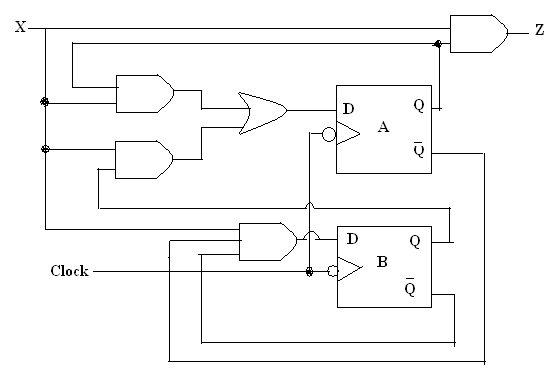Week 5 Sequential Circuit

1. State and Output Equations

To represent the output of a sequential circuit, we can use two different models as Mealy and Moore. In Mealy model, the output is a function of both present state and input. In Moore model, the output is a function of the present state only.

An example:From the circuit above, we can find the following logic equations:From the equations, we can find the state table.Now let's recap the process of converting a state table into a sequential circuit: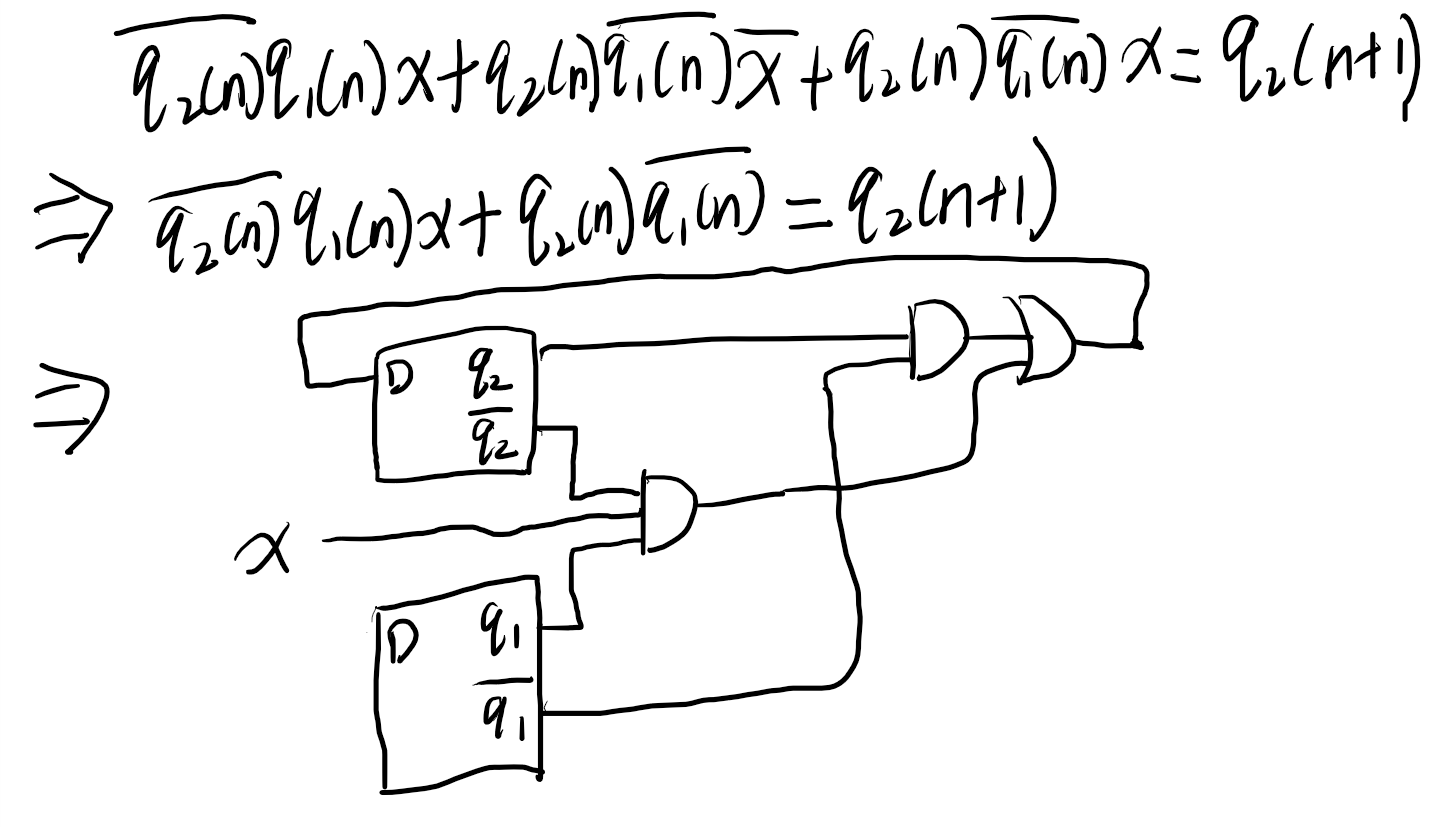You can follow the same process to find q1(n+1) and y.

2. State Diagrams

If the sequential circuit is of the Mealy type, the directed arc holds what the corresponding output will be after the state transition.The state diagram based on the Moore model requires outputs to be defined along with states. As can be seen in this figure, the directed arc only contains the input value required for transition. The circle representing the state also holds the corresponding output value.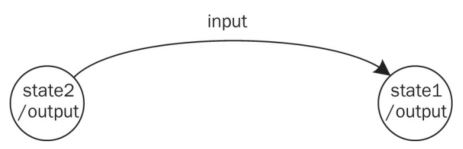The table in Section 1 is definitely a Mealy model. The state diagram is as follows: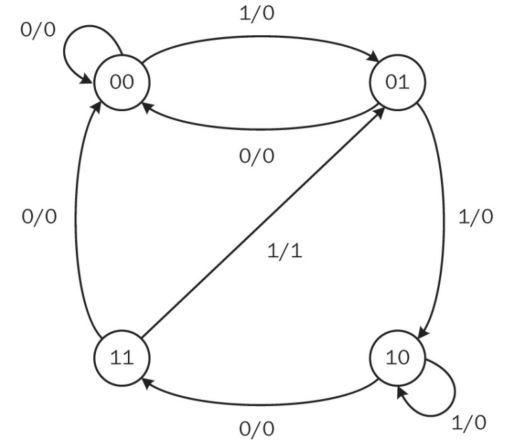3. Verilog implementation

Using the logic equations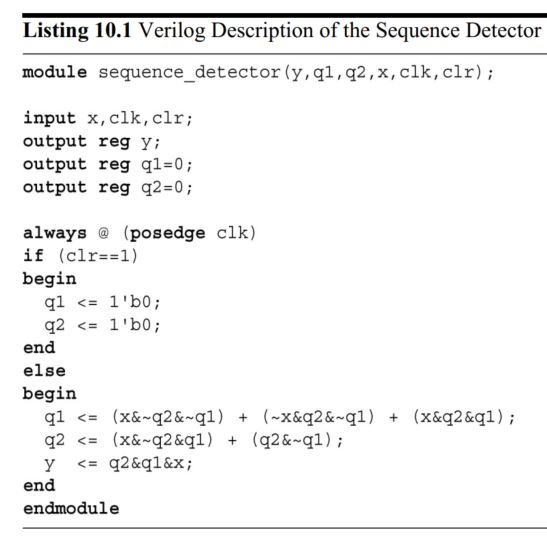Verilog implementation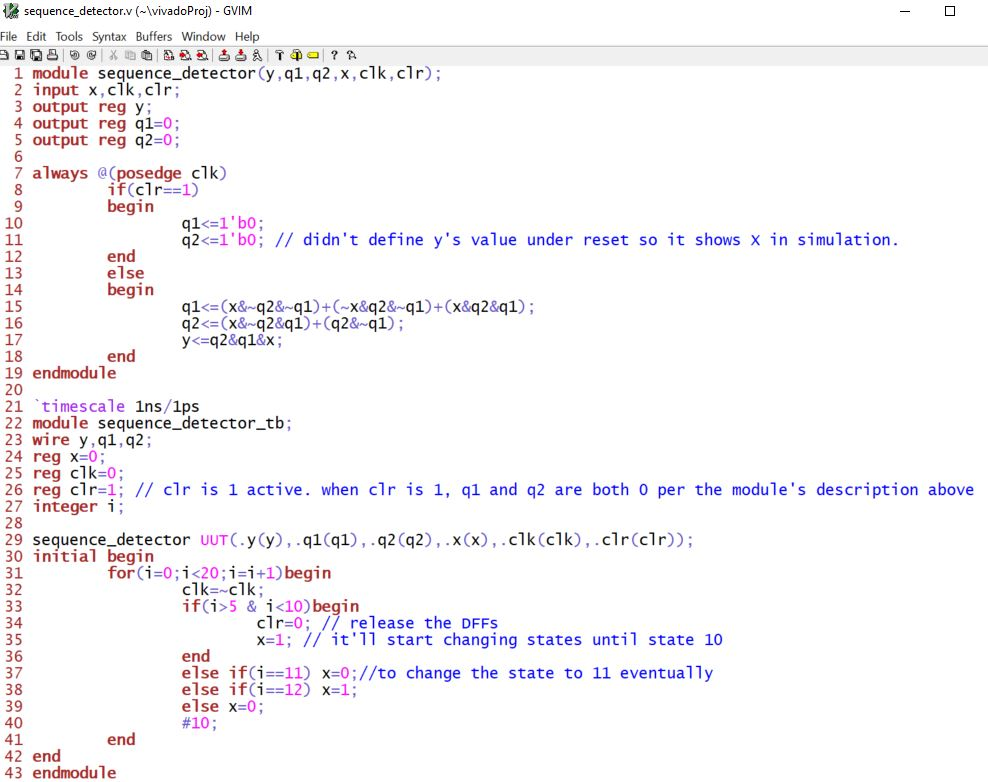When I wrote the testbench, I referred to the state diagram to find the logic states that I would like to verify. However, it didn't go through all possibilities in the state diagram. You can modify the testbench to verify all possibilities or you can run multiple simulations to verify different paths in the state diagram.

The simulation results verified the following paths in the state diagram:The rising edge of the clock is able to detect the signal change at the rising edge of X and change the state. For example at time 60ns, the rising edge detects X=1 and changed the state to q2q1 = 01, y=0.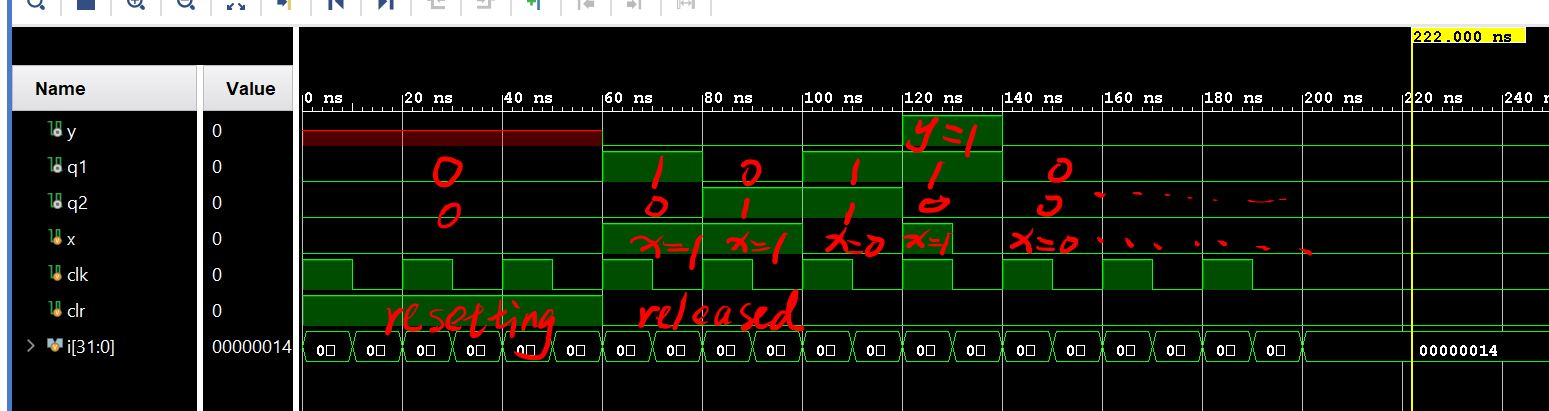Using the behavioral form

The behavioral form is nothing but a summary of the state diagram. You must include all possibilities that covered by the state diagram.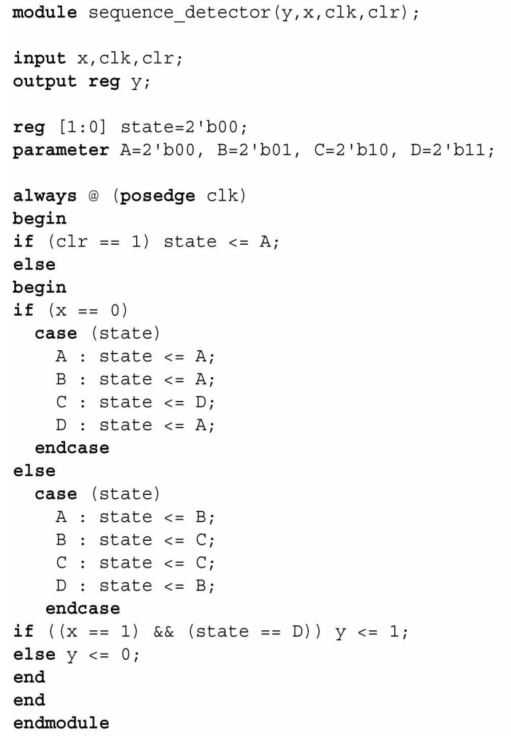Verilog script/testbench and simulation results (it shows the same simulation results to the last one.

Since we are going to test it so the 2-bit state pins should be made available for external use. The 'state' pin is declared in the module and wires are connected to the two pins from the testbench. I masked part of my script for you to fill in.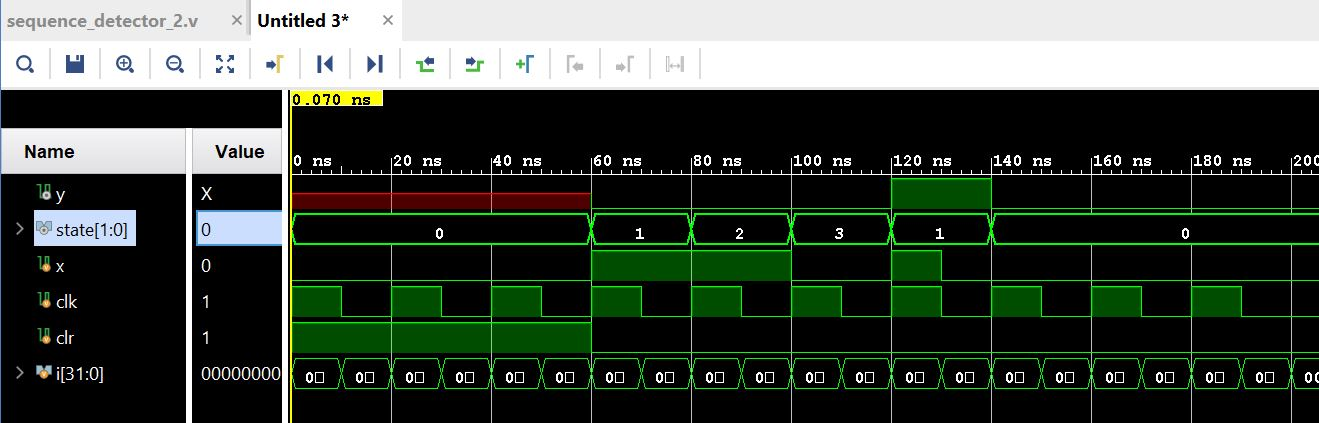4. Timing in sequential circuits

Synchronous Operation

The description under always @ (posedge clk) is responsible for state transitions and output formation. The posedge keyword indicates that the always block is executed whenever a rising edge of clock comes.

Asynchronous Operation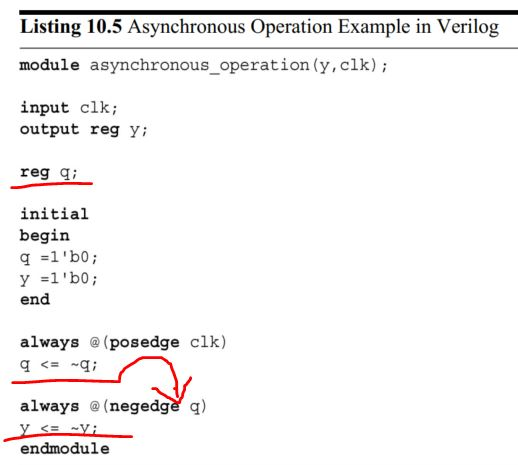5. Shift Registers

The family of devices performing this operation is called shift register. There are four shift register types: serial in/serial out, parallel in/serial out, parallel in/parallel out, and serial in/parallel out.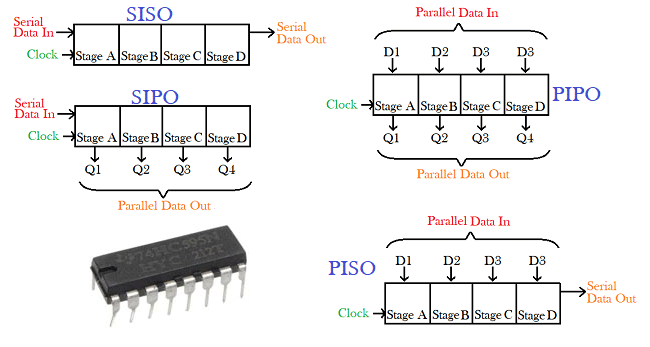Take the SISO one as a example. The circuti diagram: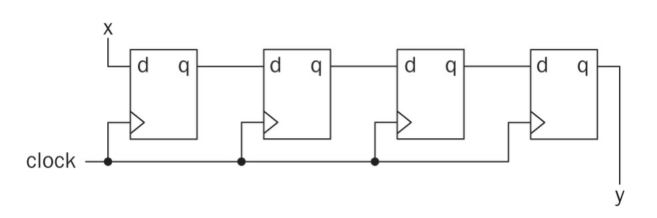Here, each bit in the sequence to be shifted is saved in a flip-flop named qi. In this setup, let q0 and qN−1 represent the least and most significant bits, respectively. This shift register can be explained best using its state and output equations as follows:As can be seen in above state equations, at every clock bits are shifted to the right flipflop. The output equation indicates this is a Moore machine since the output depends only on the present state value.

Verilog has predefined operators for shifting data in a vector. The shift right operator is “>>”. The shift left operator is “<<”. Let’s assume that a vector Q is to be shifted to left by one bit. The Verilog description for this operation will be Q << 1.

Implement SISO in Verilog: (including the testbench)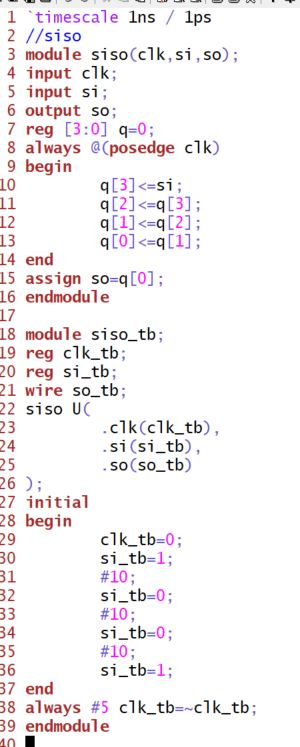The simulation results: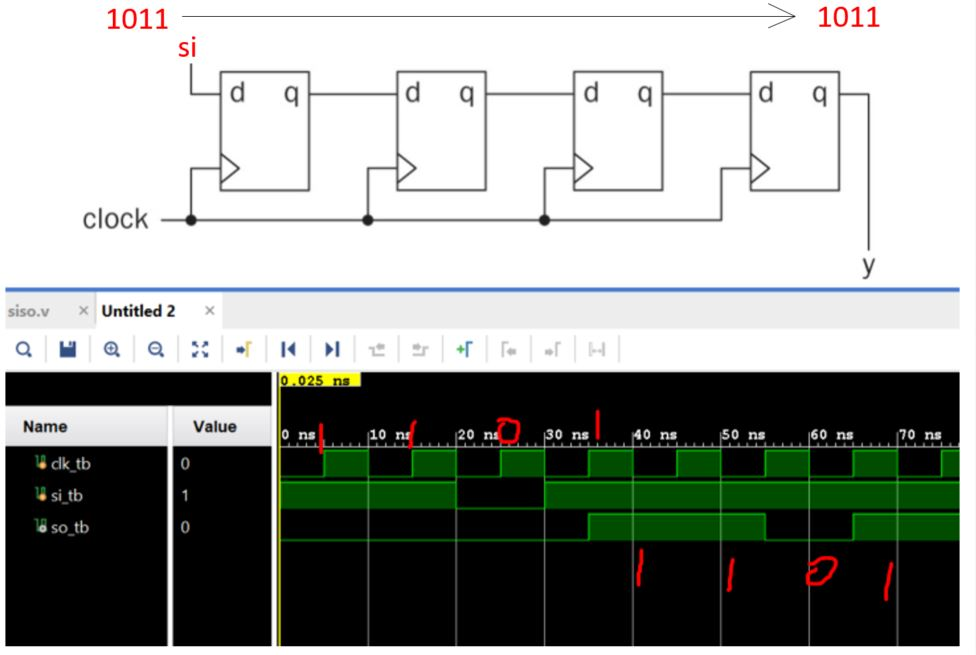Implement a right shift SIPO in Verilog (including the testbench):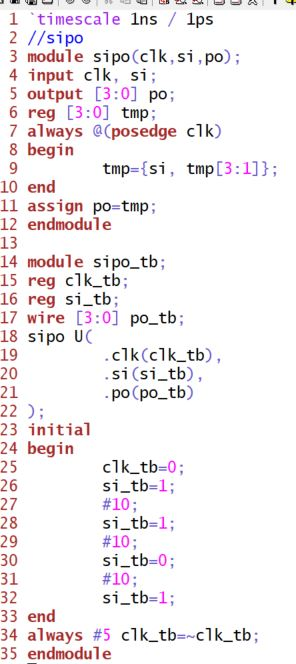The simulation results: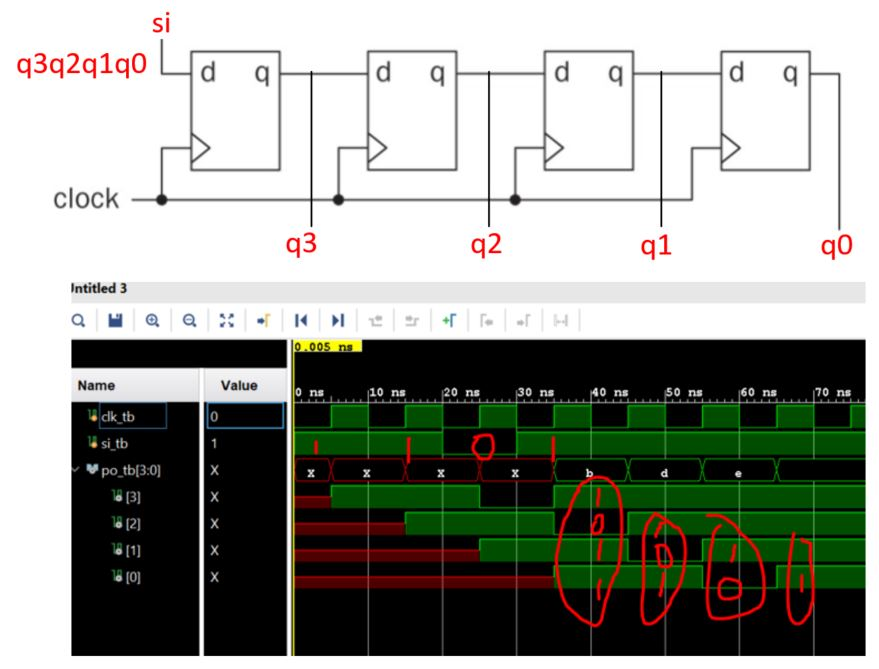PISO (parallel in serial out):

Implement it in Verilog: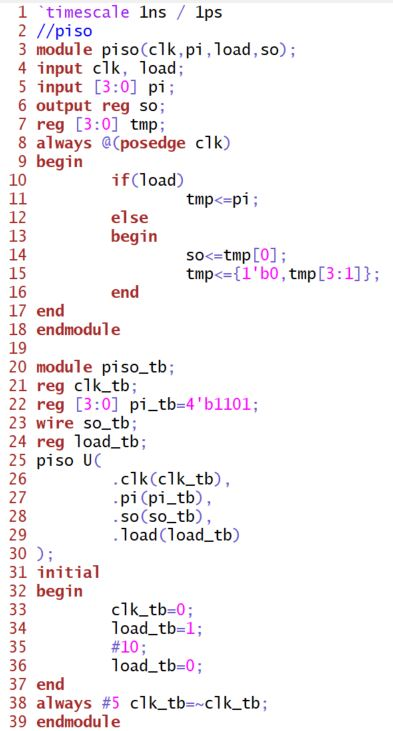The simulation results:To implement PIPO in Verilog (including the testbench):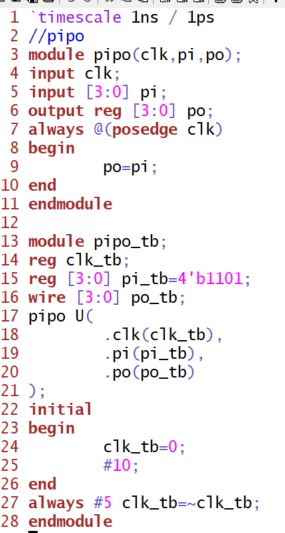The simulation results: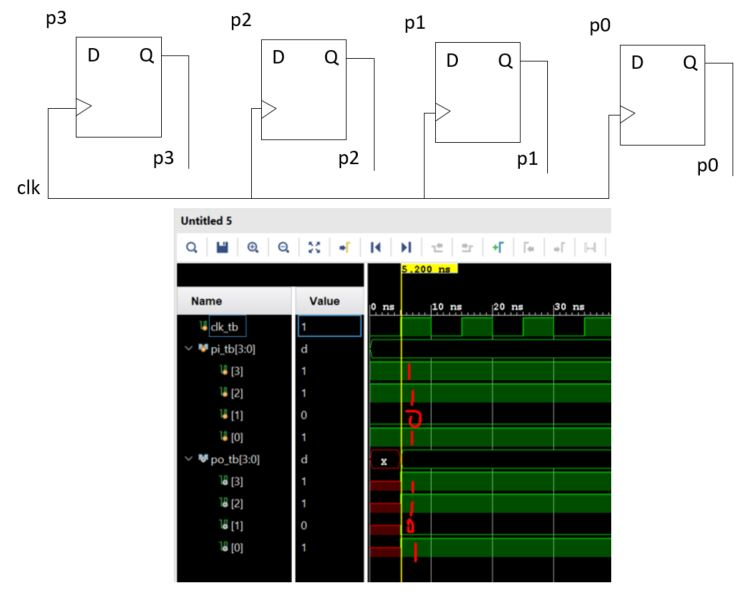6. Counter as a Sequential Circuit

The counter can best be explained by its state diagram. Let’s pick a two-bit (four state) up counter as example. States of this circuit will be 00, 01, 10, and 11. Hence, the circuit will count upwards. If the count value reaches state 11, then the next state will be 00. To indicate that the count reached the final value and restarted counting, we can set the output as logic level 1 at this transition.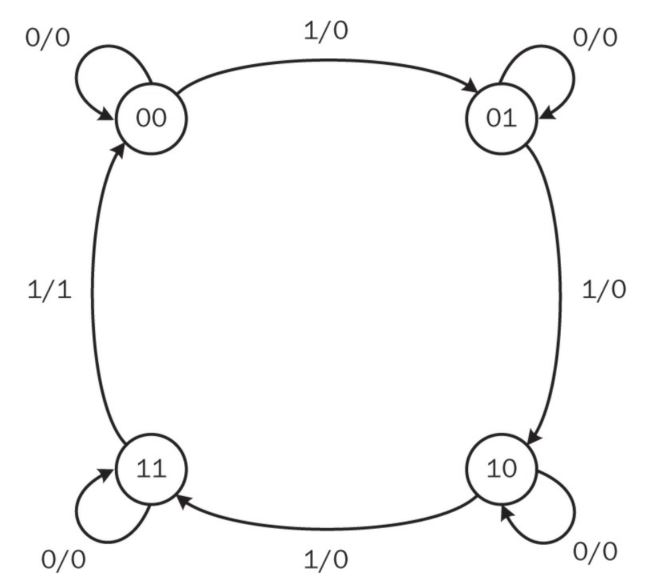A counter can be realized in two different ways as a synchronous or an asynchronous sequential circuit.

6.1 Synchronous counter

In a synchronous counter, all flip-flops within the sequential circuit are clocked with the same clock signal.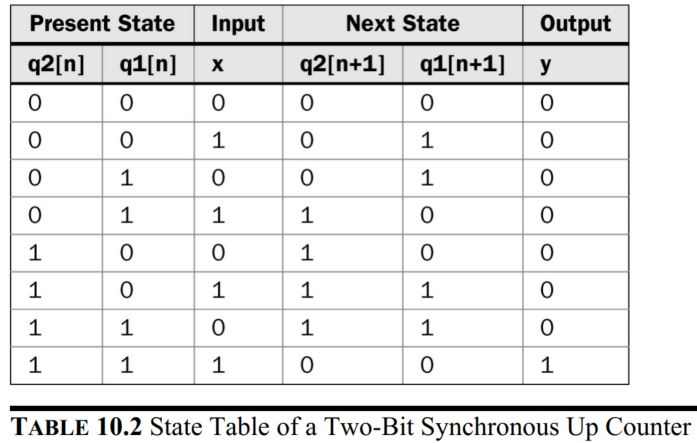The state and output equations:The sequential circuit of the 2-bit synchronous up counter: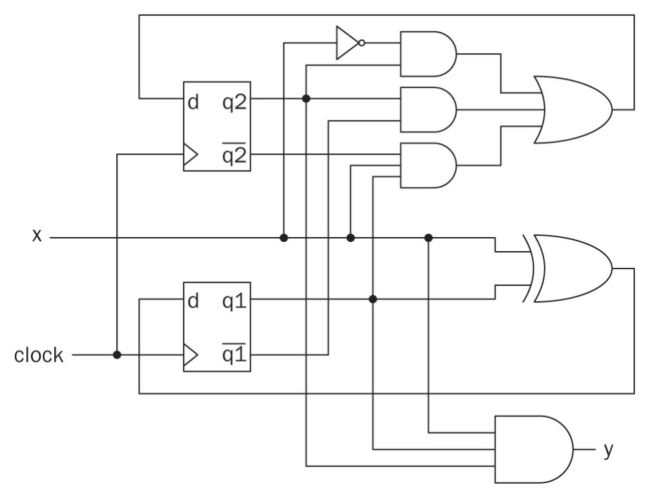In Verilog: (Please keep in mind that the {y,q}<=q+1'b1 assignment doesn't work!!! You must revise it as {y,q}<={y,q}+1'b1)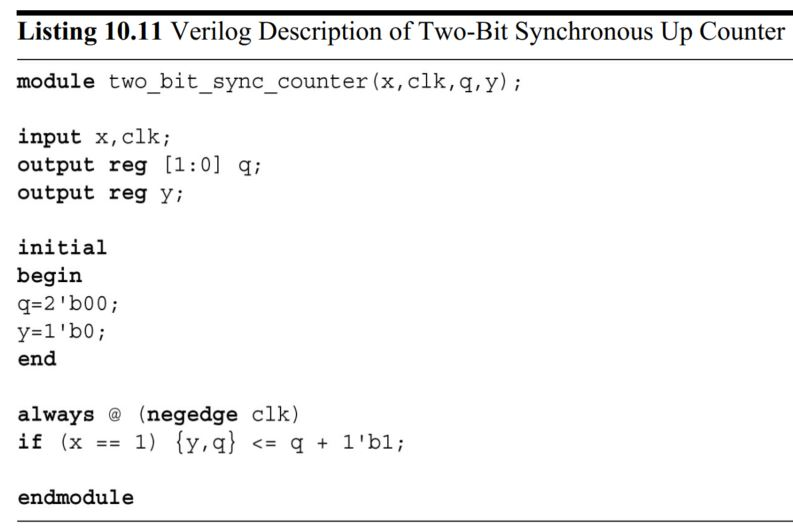An N-bit synchronous up/down counter with the 'clear' function.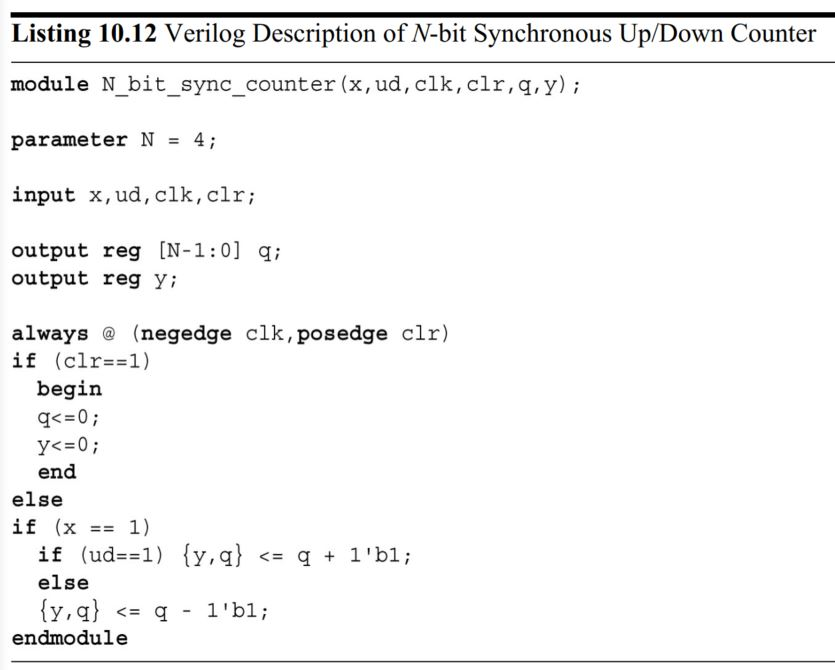6.2 Asynchronous counter

There is another way of implementing the two-bit up counter. To do so, we should analyze the state table more closely. As can be seen in this table, q1 toggles its state whenever rising edge of clock comes and input x equals to logic level 1. q2 toggles its state whenever falling edge of q1 comes and input x equals to logic level 1. This leads to asynchronous (ripple) counter in which clock signal is fed only to the first flip-flop. The second flip-flop changes its state based on the output of the first flip-flop.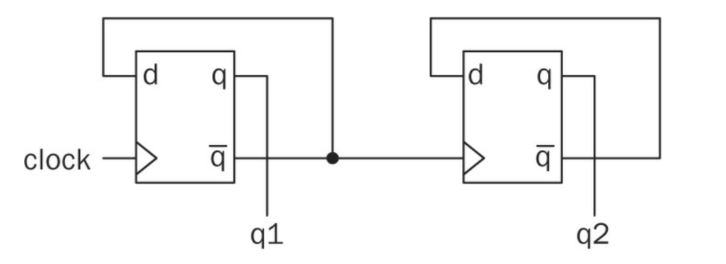7. Frequency division

The clock frequency of a digital system may not be suitable for operation. Hence, we may need to change it. Module performing this is called frequency divider. Counters can be used for this purpose. What we have to do is feeding the clock signal as inputand obtaining new clock signal with the frequency divided by powers of two from the output of counter flip-flops.

Here, we use T (toggle) flip-flops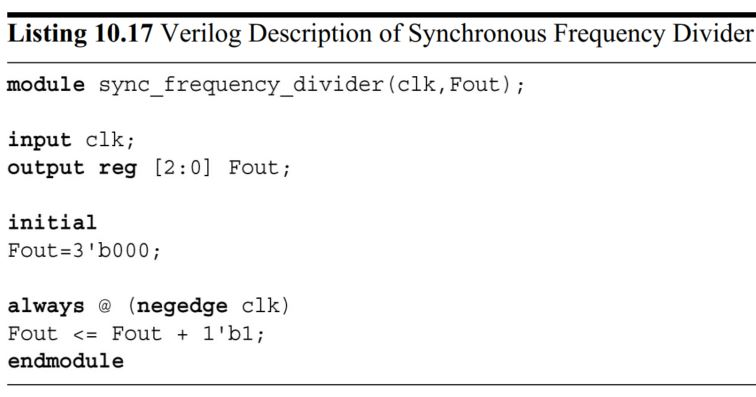--------------------------------------